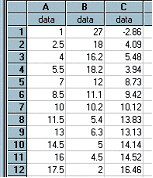﻿ PredictX(column1, column2, regtype)

# PredictX(column1, column2, regtype)

Top  Previous  Next
 column1 contains the independent variable's data (usually x values) column2 contains the dependent variable's data (usually y values) regtype is the regression model   PredictX calculates the predicted value of x for the y in column2 using the regtype regression model.   PredictX operates in exactly the same manner as Predict and all three parameters are optional.  In the example, column C has been generated using PredictX using the same data as shown for PredictY.  PredictX can predict x values for y values not in the data set in the same way that Predict can predict y values for x values not in the data set.Note:  If you type decimals using a comma (eg 3,2) you should enter this formula as PredictX(A; B; 1)  - using the ; as a separator